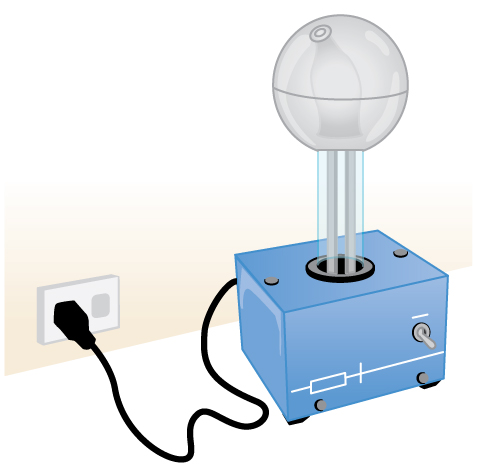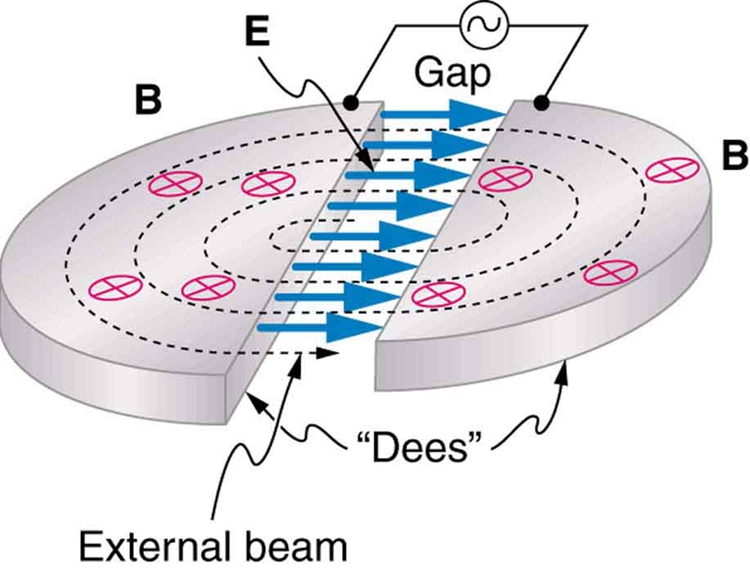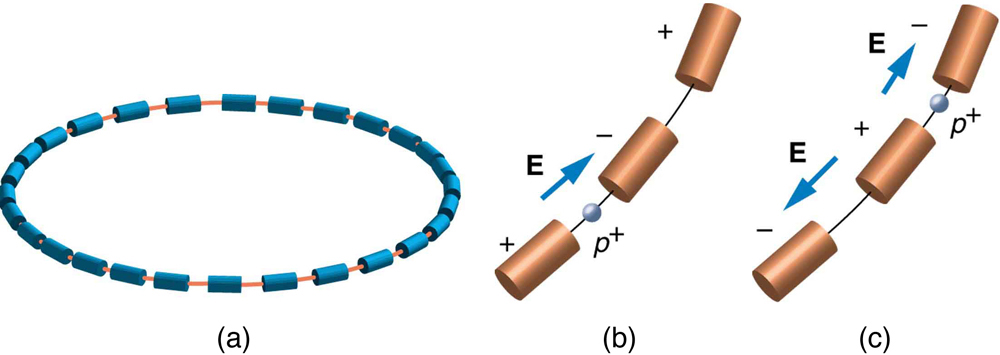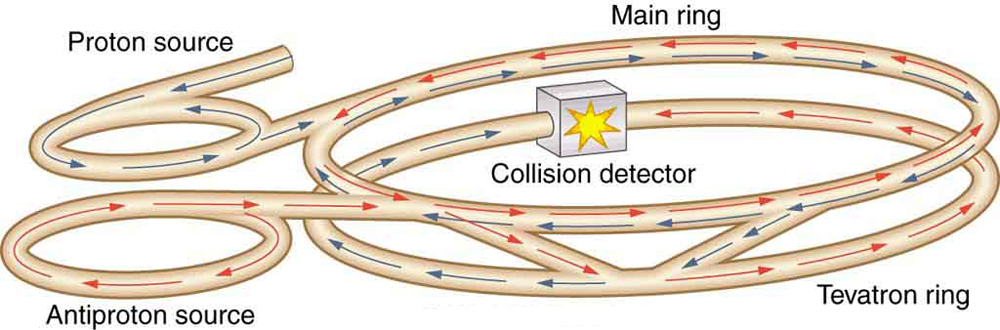# 33.3 Accelerators create matter from energy  (Page 2/9)

 Page 2 / 9An artist’s rendition of a Van de Graaff generator.Cyclotrons use a magnetic field to cause particles to move in circular orbits. As the particles pass between the plates of the Ds, the voltage across the gap is oscillated to accelerate them twice in each orbit.

## Modern behemoths and colliding beams

Physicists have built ever-larger machines, first to reduce the wavelength of the probe and obtain greater detail, then to put greater energy into collisions to create new particles. Each major energy increase brought new information, sometimes producing spectacular progress, motivating the next step. One major innovation was driven by the desire to create more massive particles. Since momentum needs to be conserved in a collision, the particles created by a beam hitting a stationary target should recoil. This means that part of the energy input goes into recoil kinetic energy, significantly limiting the fraction of the beam energy that can be converted into new particles. One solution to this problem is to have head-on collisions between particles moving in opposite directions. Colliding beams are made to meet head-on at points where massive detectors are located. Since the total incoming momentum is zero, it is possible to create particles with momenta and kinetic energies near zero. Particles with masses equivalent to twice the beam energy can thus be created. Another innovation is to create the antimatter counterpart of the beam particle, which thus has the opposite charge and circulates in the opposite direction in the same beam pipe. For a schematic representation, see [link] .(a) A synchrotron has a ring of magnets and accelerating tubes. The frequency of the accelerating voltages is increased to cause the beam particles to travel the same distance in shorter time. The magnetic field should also be increased to keep each beam burst traveling in a fixed-radius path. Limits on magnetic field strength require these machines to be very large in order to accelerate particles to very high energies. (b) A positive particle is shown in the gap between accelerating tubes. (c) While the particle passes through the tube, the potentials are reversed so that there is another acceleration at the next gap. The frequency of the reversals needs to be varied as the particle is accelerated to achieve successive accelerations in each gap.This schematic shows the two rings of Fermilab’s accelerator and the scheme for colliding protons and antiprotons (not to scale).

Detectors capable of finding the new particles in the spray of material that emerges from colliding beams are as impressive as the accelerators. While the Fermilab Tevatron had proton and antiproton beam energies of about 1 TeV, so that it can create particles up to $\text{2 TeV/}{c}^{2}$ , the Large Hadron Collider (LHC) at the European Center for Nuclear Research (CERN) has achieved beam energies of 3.5 TeV, so that it has a 7-TeV collision energy; CERN hopes to double the beam energy in 2014. The now-canceled Superconducting Super Collider was being constructed in Texas with a design energy of 20 TeV to give a 40-TeV collision energy. It was to be an oval 30 km in diameter. Its cost as well as the politics of international research funding led to its demise.

what is physics
what are the basic of physics
faith
tree physical properties of heat
tree is a type of organism that grows very tall and have a wood trunk and branches with leaves... how is that related to heat? what did you smoke man?
what are the uses of dimensional analysis
Dimensional Analysis. The study of relationships between physical quantities with the help of their dimensions and units of measurements is called dimensional analysis. We use dimensional analysis in order to convert a unit from one form to another.
Emmanuel
meaning of OE and making of the subscript nc
Negash
kinetic functional force
what is a principal wave?
A wave the movement of particles on rest position transferring energy from one place to another
Gabche
not wave. i need to know principal wave or waves.
Haider
principle wave is a superposition of wave when two or more waves meet at a point , whose amplitude is the algebraic sum of the amplitude of the waves
kindly define principal wave not principle wave (principle of super position) if u can understand my question
Haider
what is a model?
hi
Muhanned
why are electros emitted only when the frequency of the incident radiation is greater than a certain value
b/c u have to know that for emission of electron need specific amount of energy which are gain by electron for emission . if incident rays have that amount of energy electron can be emitted, otherwise no way.
Nazir
Nazir
what is ohm's law
states that electric current in a given metallic conductor is directly proportional to the potential difference applied between its end, provided that the temperature of the conductor and other physical factors such as length and cross-sectional area remains constant. mathematically V=IR
ANIEFIOK
hi
Gundala
A body travelling at a velocity of 30ms^-1 in a straight line is brought to rest by application of brakes. if it covers a distance of 100m during this period, find the retardation.
just use v^2-u^2=2as
Gundala
how often does electrolyte emits?
alhassan
just use +€^3.7°√π%-4¢•∆¥%
v^2-u^2=2as v=0,u=30,s=100 -30^2=2a*100 -900=200a a=-900/200 a=-4.5m/s^2
akinyemi
what's acceleration
The change in position of an object with respect to time
Mfizi
Acceleration is velocity all over time
Pamilerin
hi
Stephen
It's not It's the change of velocity relative to time
Laura
Velocity is the change of position relative to time
Laura
acceleration it is the rate of change in velocity with time
Stephen
acceleration is change in velocity per rate of time
Noara
what is ohm's law
Stephen
Ohm's law is related to resistance by which volatge is the multiplication of current and resistance ( U=RI)
Laura
acceleration is the rate of change. of displacement with time.
the rate of change of velocity is called acceleration
Asma
how i don understand
how do I access the Multiple Choice Questions? the button never works and the essay one doesn't either
How do you determine the magnitude of force
mass × acceleration OR Work done ÷ distance
SeemaByByBy John GabrieliBy OpenStaxBy OpenStaxBy OpenStaxBy Hannah ShethBy Tony PizurByBy Brooke DelaneyBy OpenStaxBy Stephen Voron